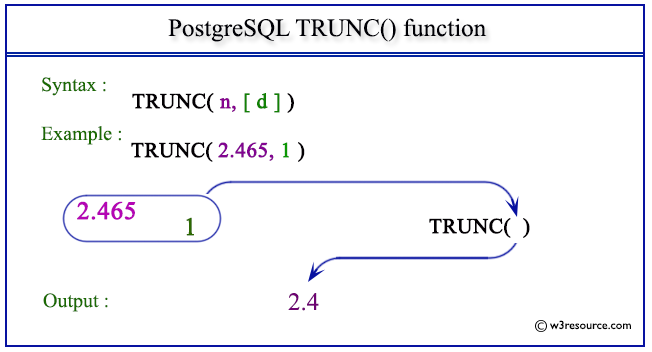# PostgreSQL TRUNC() function

## TRUNC() function

The PostgreSQL trunc() function is used to truncate a number to a particular decimal places. If no decimal places are provided it truncate toward zero(0).

Syntax:

```trunc()
```

PostgreSQL Version: 9.3

Pictorial presentation of PostgreSQL TRUNC() functionExample 1: PostgreSQL TRUNC() function

Code:

``````SELECT TRUNC(67.456) AS "Truncate";
```
```

Sample Output:

``` Truncate
----------
67
(1 row)
```

Example 2: PostgreSQL TRUNC() function

Code:

``````SELECT TRUNC(67.456,1) AS "Truncate upto 1 decimal";
```
```

Sample Output:

``` Truncate upto 1 decimal
-------------------------
67.4
(1 row)
```

Example 3: PostgreSQL TRUNC() function

Code:

``````SELECT TRUNC(67.456,2) AS "Truncate upto 2 decimal";
```
```

Sample Output:

``` Truncate upto 2 decimal
-------------------------
67.45
(1 row)
```
﻿